# CS245 Assignments 1-4 Summary

## Assignment 4

• Bottom Introduction and Negation Elimination are interchangeable.
• Solutions prefer Reflexive to Reflexivity.
• Contradiction Elimination or Negation Introduction only result in producing a formula in negation of the top one. If the top one was negated, we would resolve in double-negated form, but sometimes mistakes would occur when you subconsciously write down the negation-free form as if you applied the double negation elimination rule on that one. In this case, we could have used PBC with a range from the top, in negated form, to the contradiction, in order to avoid the mistake.
• Be careful that you must make sure you start the main proof with Premise rather than Assumption.
• If we are not allowed to use any derived rule but wishing to achieve the same performance as LEM, we could negate the conclusion and prove a contradiction. Finally, we end up using the double negation elimination rule to reach the conclusion.
• The same mistake as above. In an implication, by MT, if the conclusion if wrong, we may resolve in the negation of that hypothesis. If the hypothesis was in negation, we have to resolve in double negation, rather than mistakenly negation-free form.
• Be clear about the difference between Negation Introduction and Contradiction Elimination. By applying Contradiction Elimination, we may invent any formula but must lie within the same sub-proof. By applying Negation Introduction, however, outside the sub-proof, we resolve in the negation of the top formula of that sub-proof.
• An interesting way to prove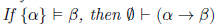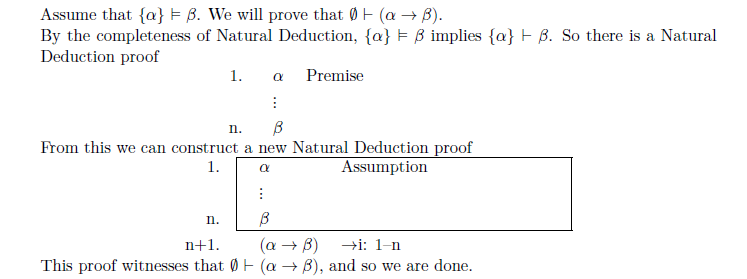• To disprove a statement ,we must exhibit an example rather than justification.

## Assignment 3

• To discuss reachability of programs in arithmetics, try to explicitly state the property, e.g. transitivity,

### Circuit Design

• Establish a corresponding truth table and focus on the rows in the truth table where the output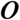is true.
• Convert each such row into a propositional formula, and then convert all four formulas together using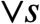.
• Assemble them into one final formula using disjunctions.

### Entailment

• To prove that an entailment holds, construct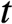as any truth valuation such that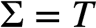.
• Try to reason that under the same, the formula to be entailed is also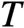.
• To prove that an entailment does NOT hold, construct a particularwith the property that under,, while the formula to be entailed is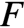under that.

• To prove that a set is an adequate set of connectives for Propositional Logic, use the fact that some set is proved an adequate set, e.g. {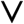,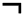} for Propositional Logic, and represent every connective in the proved set by the set to be proved.
• To prove that a set is not an adequate set of connectives for Propositional Logic, sometimes, use Structural Induction to prove that certain property of Well-formed Propositional Formulae fail to possess, e.g. failing to have negation property, i.e. every Well-formed Propositional Formula remains true under the set of connectives.

### Structural Induction

• Sometimes, only one connective case is necessary to present, and the other ones can be omitted by mentioning that they are similar to the proof of the former one.
• By Structural Induction, we can prove that certain property that holds for atoms, also, hold for Well-formed Propositional Formulae.

## Assignment 2

### Structural Induction with Prefix Involved

• In binary cases, prefixes can both be a part of formulae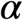and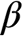. Thus, we need to discuss them case by case:
• the prefix of,
• the whole part of• the whole part ofconcatenated with the prefix of• the whole part ofand### Valuation Tree

• We might be asked to present a valuation tree in alphabetical order, since a valuation tree can be established in more than one way.
• Alternatively, every question that can be done by valuation tree can be done with truth table.

• Every formula that is a tautology is satisfiable.
•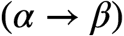cannot be a contradiction. Ifitself was a contradiction, then no matter whichwe choose, that formula is a tautology and hence, we can find ansuch that for allwe cannot form a contradiction.
• Read questions carefully. Do NOT ignore some part of question, e.g. providing an evaluation.

## Assignment 1

### Subtleties of Translating Propositions

• Conjunctions
• I will fly to Vancouver although I like taking the train.
• I am feeling very sick nonetheless I wrote my exam.
• I will go to the doctor despite not being sick.
• Although , nonetheless and despite implicitly assign conjunction to formulae.
• Only If
• I will go to work only if I am not tired.
• I go to work implies I am not tired.
• In Lieu of

• I will play hockey in lieu of playing soccer.
• I will play hockey and I won’t play soccer.
• Subscripted Propositions

• I have had dish i